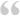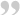Originally Said By: Jason Byrnesi cant tell anything with the code snipet you supplied.

did you also move the loop out of the function?

basically, you dont relay need to move any code, but you do need to remove the function.Sorry -- that was a little confusing.

The code snippet I posted was used to display the value that is tested in the loop.

I did move the code out of the function -- it is in it's own <?php ?> declaration now.

Here is all the PHP code in the page:

php:
``````<?php
error_reporting(E_ALL);
ini_set('display_errors','on');
?>
<?php
//WA eCart Include
require_once("WA_eCart/bookfair_PHP.php");
?>
<?php require_once('Connections/ccds.php'); ?>
<?php
\$bookfair->GetContent();
?>
<?php
if (!function_exists("GetSQLValueString")) {
function GetSQLValueString(\$theValue, \$theType, \$theDefinedValue = "", \$theNotDefinedValue = "")
{
if (PHP_VERSION < 6) {
\$theValue = get_magic_quotes_gpc() ? stripslashes(\$theValue) : \$theValue;
}

\$theValue = function_exists("mysql_real_escape_string") ? mysql_real_escape_string(\$theValue) : mysql_escape_string(\$theValue);

switch (\$theType) {
case "text":
\$theValue = (\$theValue != "") ? "'" . \$theValue . "'" : "NULL";
break;
case "long":
case "int":
\$theValue = (\$theValue != "") ? intval(\$theValue) : "NULL";
break;
case "double":
\$theValue = (\$theValue != "") ? doubleval(\$theValue) : "NULL";
break;
case "date":
\$theValue = (\$theValue != "") ? "'" . \$theValue . "'" : "NULL";
break;
case "defined":
\$theValue = (\$theValue != "") ? \$theDefinedValue : \$theNotDefinedValue;
break;
}
return \$theValue;
}
}
?>
<?php
mysql_select_db(\$database_ccds, \$ccds);
\$query_books = "SELECT * FROM books";
\$books = mysql_query(\$query_books, \$ccds) or die(mysql_error());
\$row_books = mysql_fetch_assoc(\$books);
\$totalRows_books = mysql_num_rows(\$books);

mysql_select_db(\$database_ccds, \$ccds);
\$query_orders = "SELECT * FROM orders";
\$orders = mysql_query(\$query_orders, \$ccds) or die(mysql_error());
\$row_orders = mysql_fetch_assoc(\$orders);
\$totalRows_orders = mysql_num_rows(\$orders);
?>
<?php
// WA eCart Clear Cart
if (isset(\$_POST["bookfair_Clear_1"]) || isset(\$_POST["bookfair_Clear_1_x"]))     {
\$bookfair->ClearCart();
\$Redirect_redirStr="";
if (\$Redirect_redirStr != "")     {
\$bookfair->redirStr = \$Redirect_redirStr;
}
\$bookfair->cartAction = "ClearCart";
}
?>
<?php
if (\$bookfair->IsEmpty())     {
\$bookfair->redirStr = "donationlist.php";
\$bookfair->cartAction = "RedirectIfEmpty";
}
?>
<?php
// WA eCart Redirect
if (\$bookfair->redirStr != "")     {
}
?>

<?php
\$now=mktime();
do    {
if (\$bookfair->ConditionalTotal("Quantity", "recID", \$row_books['recID']) > 0) {
\$query = mysql_query('UPDATE books SET purchaseDate = '.\$now.' WHERE recID = '.\$row_books['recID']);
}
}
while (\$row_books = mysql_fetch_assoc('books'));
?>``````

The snippet I previously posted is in the <body> of the page, just to display the result of the if statement. . .